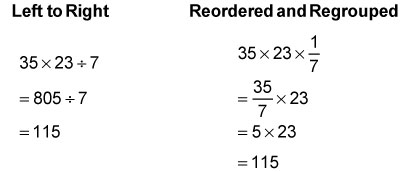##### ASVAB AFQT For Dummies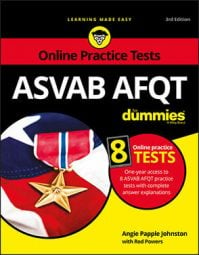Anything that saves you time and brain power on the ASVAB AFQT Mathematics Knowledge subtest is useful for two reasons: first, because you're working on a limited time budget, and second, because you can't use a calculator. That's where math properties, like the commutative and associative properties, can help.

The commutative and associative properties let you break the rules about adding or multiplying from left to right:

• The commutative property of addition says you can rearrange the numbers you're adding without changing the result.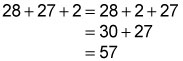• Similarly, the associative property of addition lets you decide how to group the numbers you're adding.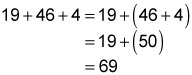Together, these properties let you add a string of numbers in whatever order you like. For example, you can make calculations easier by pairing up numbers whose ones digits add up to 10 before adding other numbers in the list.

Because subtracting is essentially the same thing as adding a negative number, you can extend these addition properties to subtraction problems, too — just be careful to keep track of the negative signs. The following example shows how smart groupings can let you add and subtract figures faster. Notice which calculations are easier to do in your head.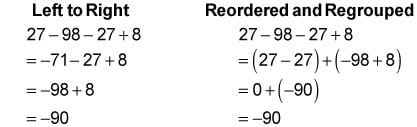Similarly, the commutative and associative properties of multiplication let you multiply numbers in any order you like. Check out how switching the numbers around can make mental math easier: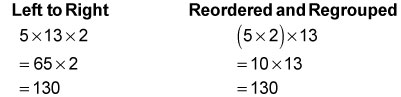You can even use these multiplication properties with division, as long as you remember that division is the same thing as multiplying by a fraction: